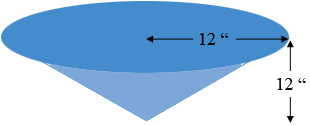SEARCH HOMEMath Central Quandaries & QueriesQuestion from Neil, a parent: I had a 24 foot diameter pool. The perimeter of it was at ground level. The pool sloped 1 foot deeper to the middle. In other words "a 1 ft. dish. How many cubic yards of dirt do I need to fill this hole?Hi Neil,

Your pool has the shape of a cone. Here is my diagram, not to scale.The volume of a cone is $\frac13 \pi\; r^2 h$ where $r$ is the radius, $h$ is the height, and $\pi$ is approximately 3. 1416. This will give you an answer of $V$ cubic feet. To determine the volume in cubic yards use Goggle. Once you know the value of V type into the Goggle search window V cubic feet in cubic yards.

PennyMath Central is supported by the University of Regina and The Pacific Institute for the Mathematical Sciences.